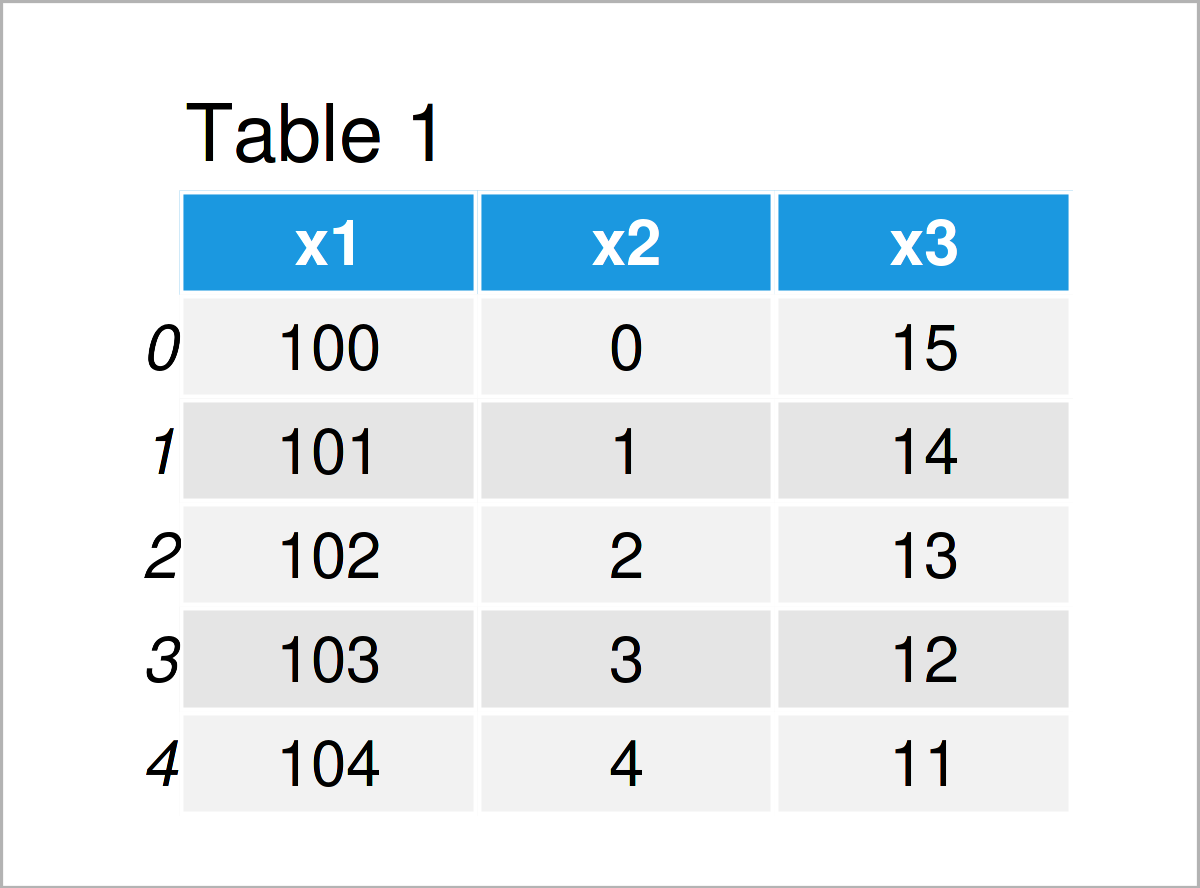# Iterate Over Columns of pandas DataFrame in Python (2 Examples)

In this tutorial you’ll learn how to iterate through the columns of a pandas DataFrame in the Python programming language.

The content of the post looks as follows:

Here’s the step-by-step process…

## Example Data & Libraries

To be able to use the functions of the pandas library, we first have to load pandas.

`import pandas as pd                           # Import pandas library to Python`

Let’s also create some example data in Python:

```data = pd.DataFrame({'x1':range(100, 105),    # Create pandas DataFrame
'x2':range(0, 5),
'x3':range(15, 10, - 1)})
print(data)                                   # Print pandas DataFrame```Table 1 shows that the example pandas DataFrame comprises five rows and three columns.

## Example 1: Use for Loop to Iterate Over Columns of pandas DataFrame

The Python code below demonstrates how to iterate over the columns of a pandas DataFrame in Python.

In this specific example, we will convert the variable that corresponds to the iteration to a list and print its values within a well-formatted sentence.

Consider the Python code below. As you can see, it consists of two lines of code. The first line defines the loos, and the second line defines the tasks for each iteration of this loop.

Let’s apply our loop:

```for column in data:                           # Print values of each column
print('The values of this column are', data[column].tolist())
# The values of this column are [100, 101, 102, 103, 104]
# The values of this column are [0, 1, 2, 3, 4]
# The values of this column are [15, 14, 13, 12, 11]```

As you can see, the execution of the previous Python code has returned one sentence for each column of our data set.

## Example 2: Perform Calculation by Column within for Loop

Example 1 has shown how to print the values and some text within a for loop.

In Example 2, I’ll explain how to conduct some calculations within each iteration of a loop.

More precisely, this example calculates the sum of a column and then prints the result to the Python console:

```for column in data:                           # Calculate sum of each column
print(sum(data[column]))
# 510
# 10
# 65```

The previous Python syntax has returned the sum for each column of our pandas DataFrame.

Please note that results like this can often be easier to achieve with other Python codes, and loops are often not the best solution. In case you want to learn more about the advantages and disadvantages of for loops in Python, you may have a look here.

## Video & Further Resources

I have recently released a video tutorial on my YouTube channel, which illustrates the topics of this article. You can find the video tutorial below.

Please accept YouTube cookies to play this video. By accepting you will be accessing content from YouTube, a service provided by an external third party.If you accept this notice, your choice will be saved and the page will refresh.

In addition, you may read the other tutorials on my website. I have released several related articles that are related to the iteration through the columns of a pandas DataFrame already:

This article has explained how to loop and enumerate through the columns of a pandas DataFrame in Python. Let me know in the comments below, if you have further questions.

Subscribe to the Statistics Globe Newsletter